# Remote Plant Monitor

Simple IoT project with two Particle Argons. One measures soil moisture, the other raises an alert flag.

BeginnerFull instructions provided2 hours158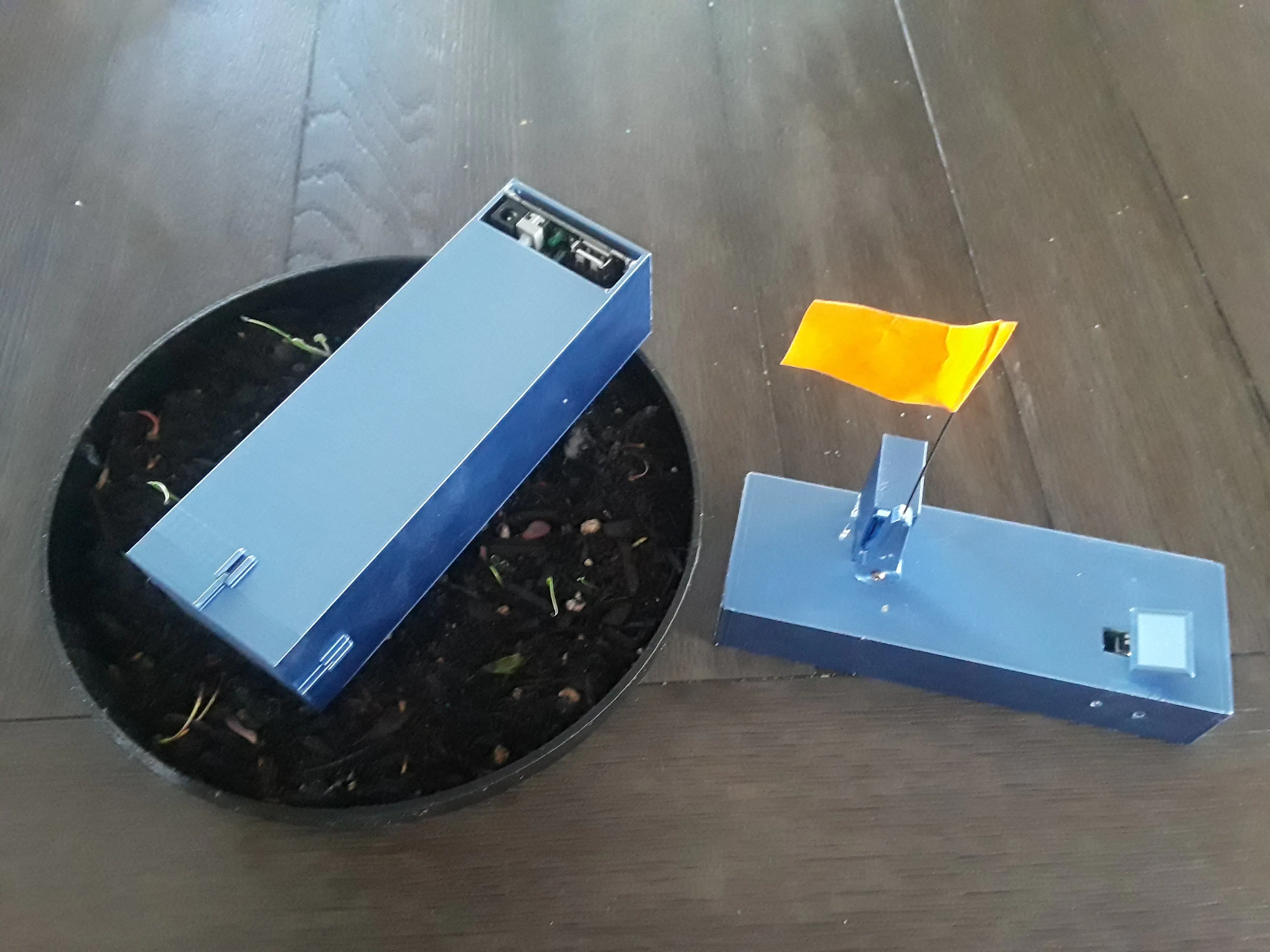## Things used in this project

### Hardware components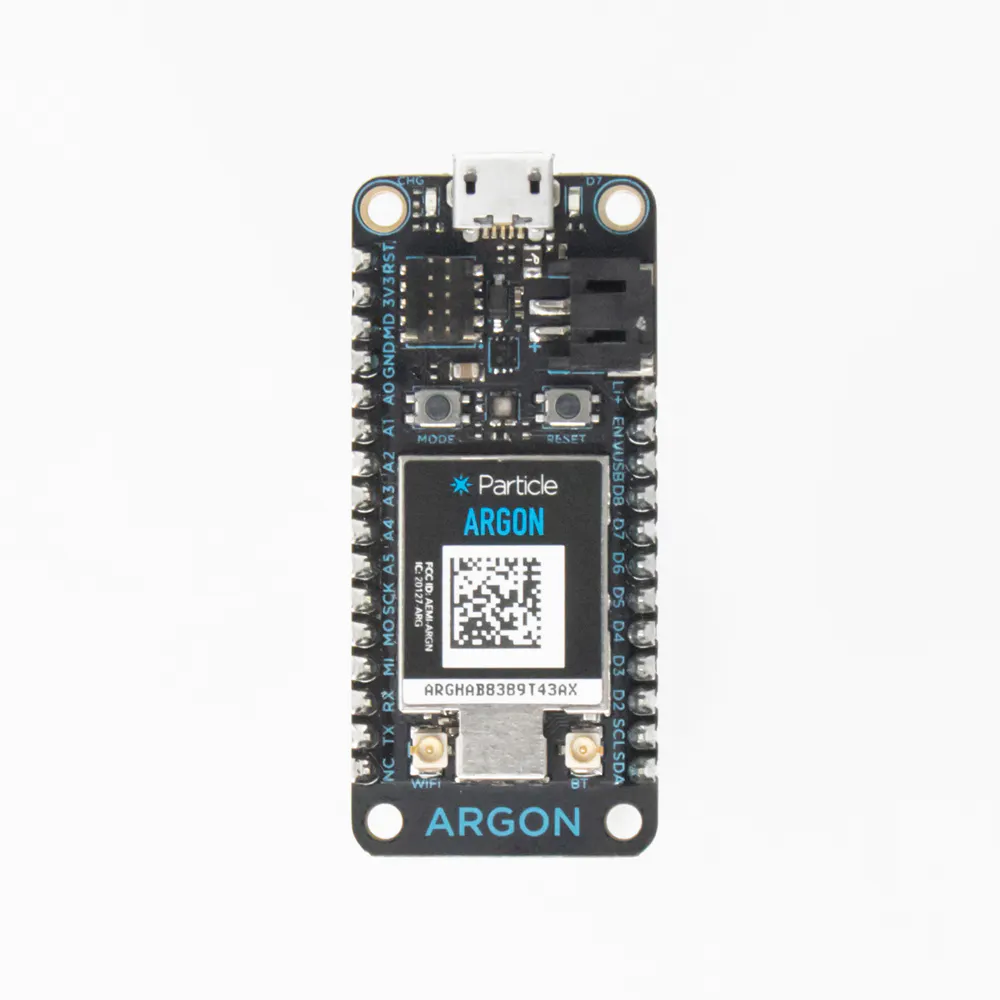Particle Argon
×2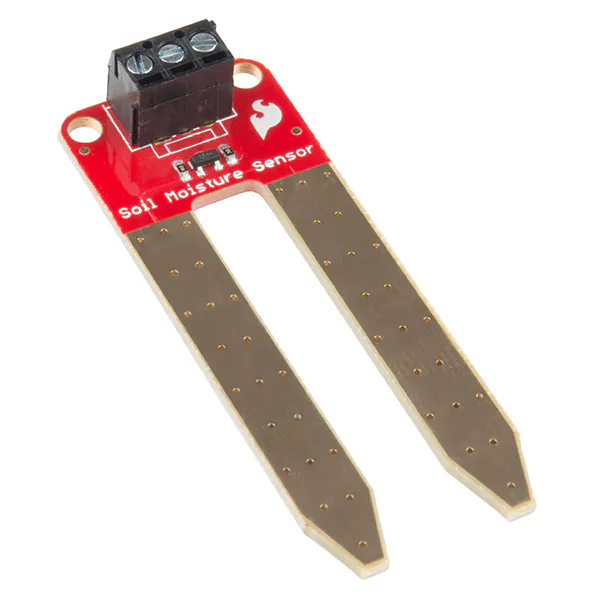SparkFun Soil Moisture Sensor (with Screw Terminals)
×1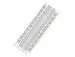×2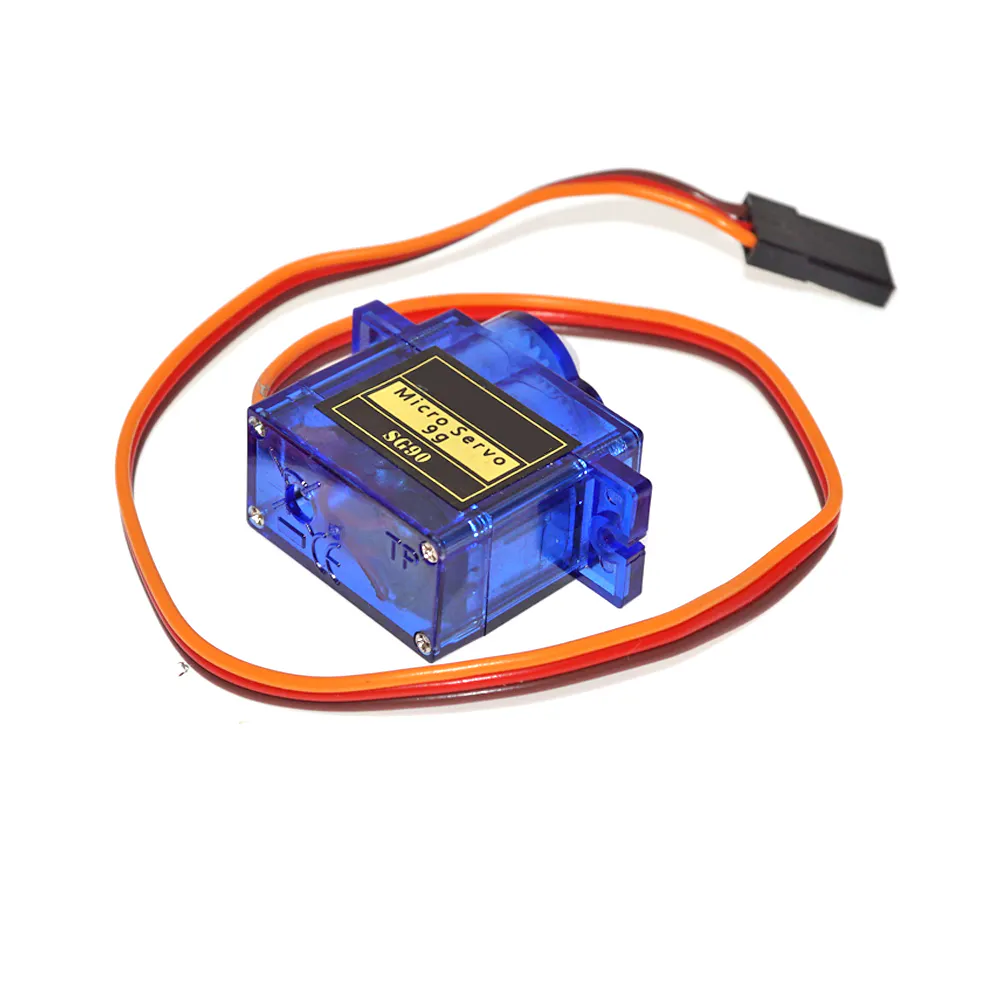SG90 Micro-servo motor
×1
 Mechanical Endstop Limit Switch
×1
×2
 12V DC power adapter 5.5mm x 2.1mm barrel plug
×2
 3D Printed Casing
×2

### Software apps and online servicesParticle Build Web IDEThingSpeak API
 Fritzing Circuit Design
 Solidworks

### Hand tools and fabrication machines3D Printer (generic)

## Custom parts and enclosures

### Moisture Assembly Parts

Parts in STL form. PSU mount requires cutting/desoldering PSU breadboard pins.

Parts in STL form. Limit switch mount uses M2 cap head screws.

## Schematics

### Moisture Sensor Argon Wiring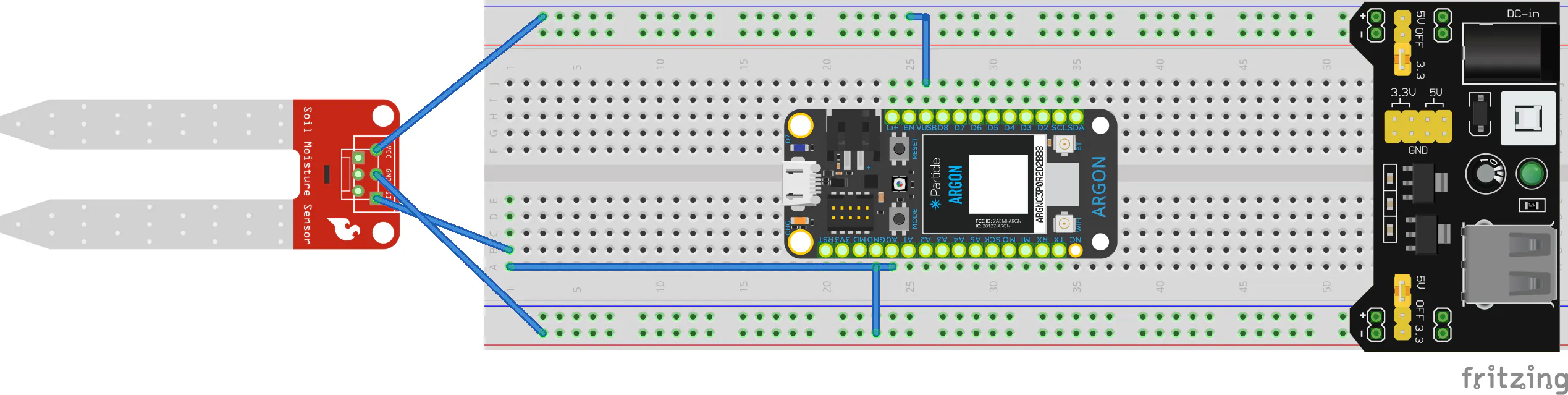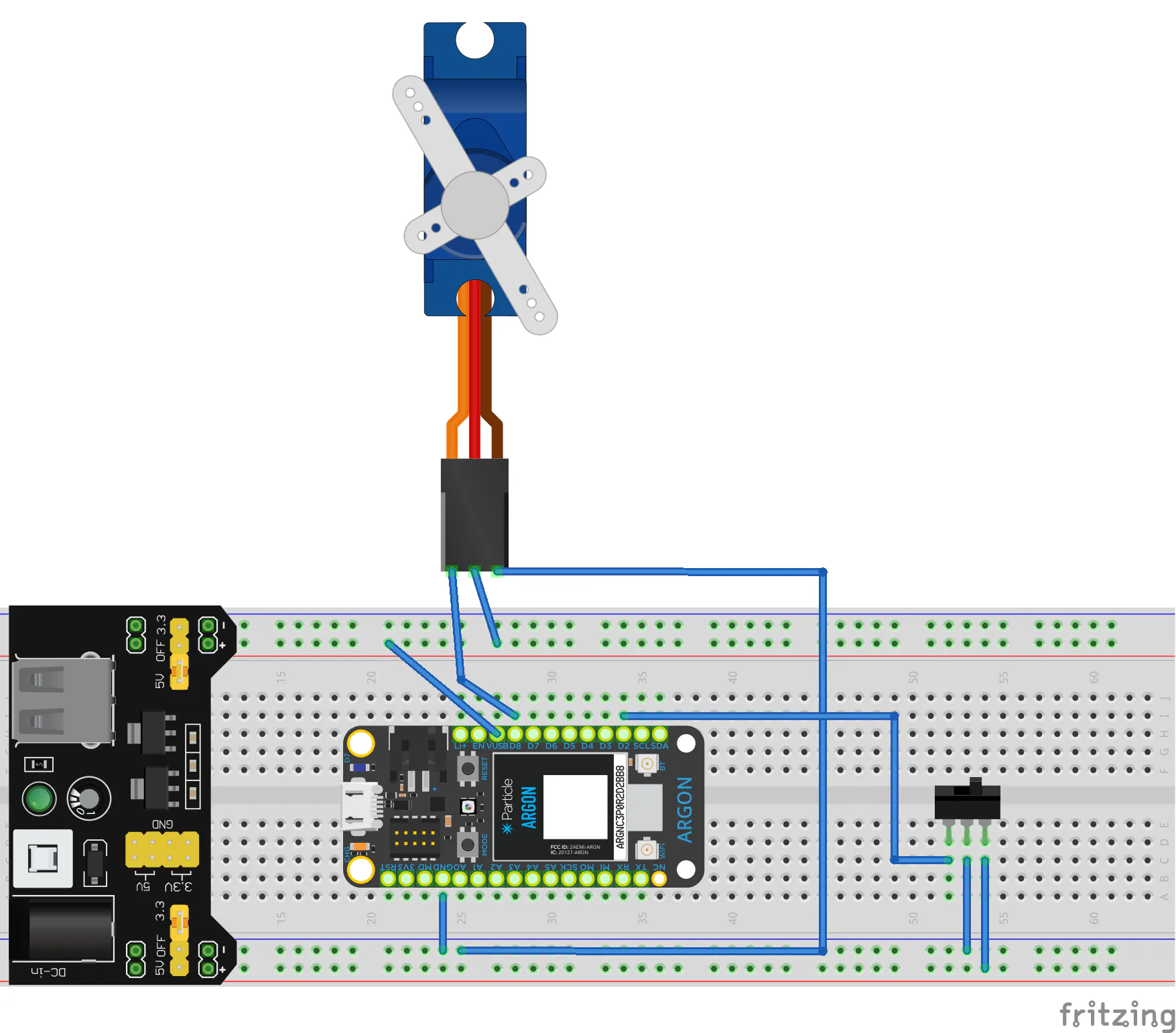## Code

### final-send-long.ino

C/C++
Code of the moisture sensor argon
```int threshold = 3130; //sensor value where soil is considered dry
int dry = 0;//
int count_1 = 4000; //setting inital values for the stored sensor values well above threshold to stop the dry flag from triggering of the inital values
int count_2 = 4000; // each of these counts represent a moisture sensor value for one loop
int count_3 = 4000; // each loop, the current sesor calues is moved to the next count and the next sensor value is assigned to count_1
int count_4 = 4000;
int count_5 = 4000;
int moisture_percent = 100;
int num_under = 0; // number of the past 5 sensor values that are under the  dry threshold

void setup() {//Runs once upon initialization
pinMode(18, INPUT);// Connect Moisture sensor to A1
Particle.subscribe("restart", restart);// listen for restart flag from other argon
// when flag is recieved
}

void loop() {//Runs Repeatedly
num_under = 0;//resets the number of sensor values under
count_5 = count_4;  // Assign the previous sensor value to the next count.
count_4 = count_3;  // Ex . [1300, 4000, 40000, 0, 0] --> [1300, 1300, 0, 0, 0]
count_3 = count_2;
count_2 = count_1;
count_1 =  analogRead(18); // Read sensor  value and assign it to count 1 Ex. [1400, 1300]
moisture_percent = 100 * count_1 / threshold;
Particle.publish("moisture sensor", String(moisture_percent));
delay(100);

if (dry == 0){ // only runs if the dry flag is not activated
if (count_1 <= threshold){ // counts the number of sensor values under threshold
num_under = num_under + 1; // Ex count 1 less than or equal to threshold --> num_under increaces by one
}
if (count_2 <= threshold){
num_under = num_under + 1;
}
if (count_3 <= threshold){
num_under = num_under + 1;
}
if (count_4 <= threshold){
num_under = num_under + 1;
}
if (count_5 <= threshold){
num_under = num_under + 1;
}
if (num_under >= 3){ // if the total number of sensor values under threshold is greater than or equal to 3...
dry = 1;         // the dry flag is set to 1 (true)
}
}

Particle.publish("Dry flag", String(dry));
// the status of the dry flag is always sent to the other argon every loop so if the other argon is
// not conncted when the flag switchs from false (0) to true (1), the other argon will still activate the servo nofification

delay(3600000); // 10 sec loop time

}

void restart(const char *event, String data){ // Runs only when the restart flag is updated by the other argon which is when the flag is detect to be placed in the down position

dry = 0; //sets the dry flag to false(0)
//the argon will continue counting sensor values under threshold with dry set back to false
count_1 = 4000;
count_2 = 4000;
count_3 = 4000;
count_4 = 4000;
count_5 = 4000;
}
```

### final-recieve-long.ino

C/C++
```Servo myservo;
int servo_up = 0; // a True/False variable indication of if the servo should be in the up or down position
int cycle = 0; // number of cycles for

void setup() {
pinMode(8, INPUT); // pin D8 limit switch wiring
myservo.attach(A1);// servo pwm connected to in A1
Particle.subscribe("Dry flag", servoup); //listen for the dry flag to be updated then run the servoup function
}

void loop() {
int restartinv =  digitalRead(8); // for the button I'm using to test, 0 is pressed and 1 is unpressed
int restart;                      // uninverted restart variable
if (restartinv == 0){ // these if fuctions convert the inverted restart variable to the expected 1 == true and 0 == false
restart = 1;
}
if (restartinv == 1){
restart = 0;
}

cycle = cycle + 1; // redundancy if the flag is moved down or up unintentionally
// we do not want the servo to move back to its intended position every loop so
if (cycle == 3600){  // this function only runs every n number of cycles (activates every hour)
cycle = 0; // restart cycle number
if (servo_up == 0){ // if servo is suppose to be down, move the servo down
myservo.attach(A1); // attach the servo the A1
myservo.write(10); //move the servo position down
delay(1000); // allow 1 sec for the servo to move
myservo.detach(); // diconnect the servo so it can be moved by hand
}
if (servo_up == 1){ // repeat of previous loop but for the up position
myservo.attach(A1);
myservo.write(90);
delay(1000);
myservo.detach();
}
}
if (restart == 1){//this part of the code sends the signal for the other argon to look for dry soil again and move the notification servo up
delay(5000); //delay to allow the user to be clear from the servo before it moves
Particle.publish("restart", String(restart)); // send the restart flag to the other argon
myservo.attach(A1);    // moving the servo
myservo.write(10);     // set servo to a positon slightly above the switch to indicate the reset is recieved
delay(1000);           // allow time for the servo to move
myservo.detach();      // diconnect the servo so it can be moved by hand
servo_up = 0;          // sets servo position to down
delay(20000);          // allows dry flag to reset on the other argon before resuming looking for button press will need to be modified if the other argons loop time is increaced
}
delay(1000);// changes loop time, about 1 sec
}

void servoup(const char *event, String data){
int dry = String(data).toInt(); // converting the published string to an integer
if (dry == 1 && servo_up == 0 ){// if the other argon says the soil is dry and the servo is down
myservo.attach(A1);// put the servo up
myservo.write(90);
delay(1000);
myservo.detach();
servo_up = 1;
}
}
```

## Credits

### cody davis

1 project • 0 followers

### Krishna Mathavan

1 project • 0 followers